# SAT II Math II : Graphing Trigonometric Functions

## Example Questions

### Example Question #293 : Sat Subject Test In Math Ii

Give the amplitude of the graph of the function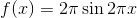Explanation:

The amplitude of the graph of a sine function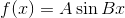is. Here,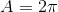, so this is the amplitude.

### Example Question #294 : Sat Subject Test In Math Ii

Which of these functions has a graph with amplitude 4?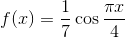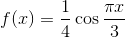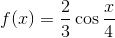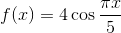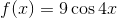Explanation:

The functions in each of the choices take the form of a cosine function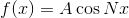.

The graph of a cosine function in this form has amplitude. Therefore, for this function to have amplitude 4,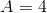. Of the five choices, onlymatches this description.

### Example Question #11 : Graphing Functions

Which of these functions has a graph with amplitude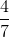?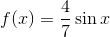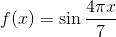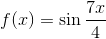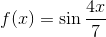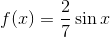Explanation:

The functions in each of the choices take the form of a sine function.

The graph of a sine function in this form has amplitude. Therefore, for this function to have amplitude 4,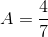. Of the five choices, onlymatches this description.

### Example Question #296 : Sat Subject Test In Math Ii

Which of the following sine functions has a graph with period of 7?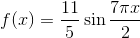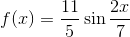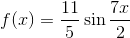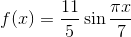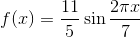Explanation:

The period of the graph of a sine function, is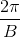, or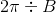.

Therefore, we solve for: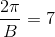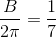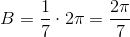The correct choice is therefore.

### Example Question #8 : Period And Amplitude

Which of the given functions has the greatest amplitude?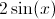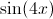The amplitude of a function is the amount by which the graph of the function travels above and below its midline. When graphing a sine function, the value of the amplitude is equivalent to the value of the coefficient of the sine. Similarly, the coefficient associated with the x-value is related to the function's period. The largest coefficient associated with the sine in the provided functions is 2; therefore the correct answer is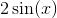.